Related Articles

# Plot Expression or Function in MATLAB

• Last Updated : 28 Apr, 2021

In this article, we will discuss how to plot expressions or functions in MATLAB. We can make use fplot() function in MATLAB to generate the plot corresponding to an expression or function.

There are different variants of fplot() function

1. fplot(f)
2. fplot(f,xinterval)
3. fplot(___,LineSpec)
4. fplot(___,Name,Value)

Now discussing each variant in detail. Below are the various ways to plot an expression or a function in MATLAB:

fplot(f): Plots the expression passed to it as a parameter.

Example : Plotting cos(x) function in default interval [-5 5]

## Matlab

 `% Plots cos(x) function from x=-5 to 5``fplot(@(x) cos(x))`

Output :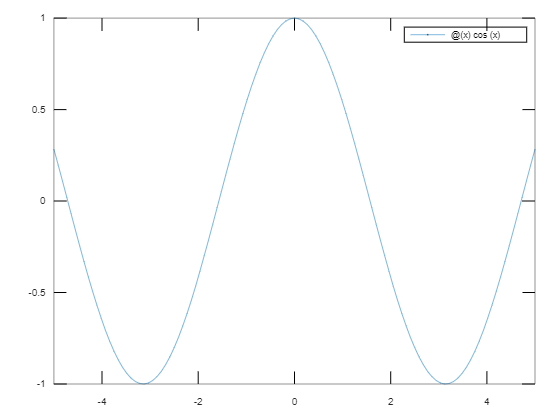fplot(f,xinterval): Plots the curve defined by function y = f(x) in the specified interval. Specify the interval as a two-element vector of the form [xmin xmax].

Example : Plotting cos(x) function in the interval [-3 3].

## Matlab

 `% Plots the sin(x) cureve from x =[-3:3]``fplot(@(x) sin(x),[-3 3])`

Output :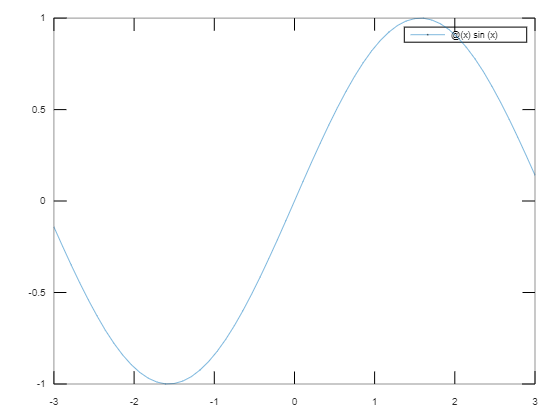fplot(___,LineSpec)

• It allows specifying the line properties such as line style, marker symbol, and color.
• For example, ‘b’ plots a blue line. We can specify these properties after specifying the function and the interval.
• Line style, marker, and color, specified as a character vector or string containing symbols in any order. For example: ‘-*r’ means red solid line with Asterisk. You can also omit some properties.

Some values of each property are

Example : Plotting exp(x) function in the interval [-5 8] with a red solid line with star marker.

## Matlab

 `% plotting exp(x) function in the interval [-5 8] ``% with a red solid line with star marker.``fplot(@(x) exp(x),[-5 8],``'-*r'``)`

Output :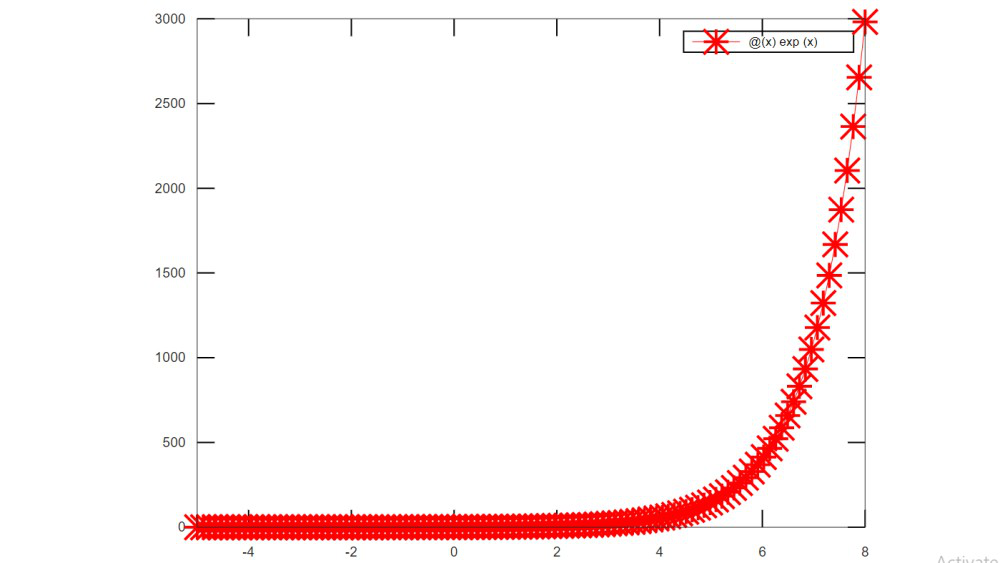fplot(___,Name,Value)

• Another way to specify the properties of line with Name-Value pair of arguments.
• For example ‘Color’, ‘r’ indicated the red line.
• We can also specify properties such as LineWidth, Marker, Color, LineStyle.

Example : Plot the sin(2x) function with a linewidth of 2, and blue dotted line with a circle marker by specifying their name and value pairs.

## Matlab

 `% Plot the sin(2x) function with a ``% linewidth of 2, and blue dotted ``% line with circle marker``fplot(@(x) sin(2*x),[-5 8],``'Color'``,``'b'``,``'Marker'``,``'o'``,``'LineWidth'``,2,``'LineStyle'``,``'--'``)`

Output :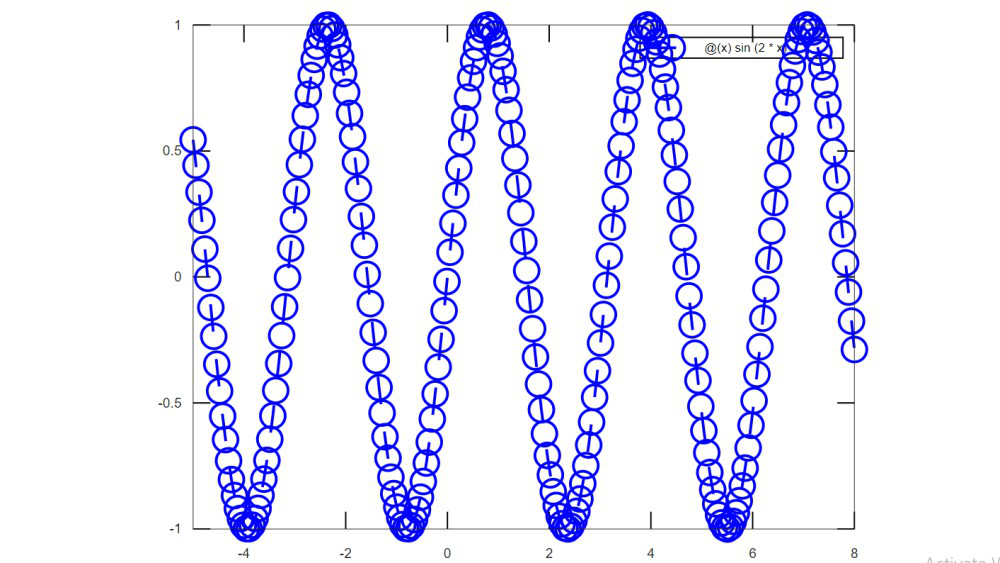We can depict multiple expressions in a single plot. Below is an example where we plot sin(x) and cos(2*x) in the same illustration:

## Matlab

 `% Plotting sin(x)``fplot(@(x) sin(x))``hold on `` ` `% Resuming the plot and ``% including cos(2*x)``fplot(@(x) cos(2*x))``hold off`

Output :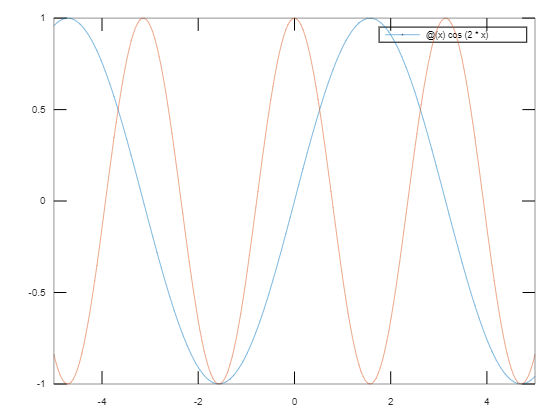In the above program, we depict two expressions in the same plot. The hold keyword is used in MATLAB to hold on and hold off expressions in a plot.

My Personal Notes arrow_drop_up